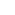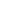For Customised Solutions :+91-7541867385 |Contact UsMathematics is one of the most important subjects taught to students in school and it has applications in a variety of disciplines. Equations are a very important part of learning mathematics. Mathematical equations are like math problems that need to be analyzed and then solved using various formulae and mathematical techniques.

It’s very important for teachers to test their students’ understanding of math equations before they can go on to explaining more complex mathematical concepts. An assessment for math equations is a great way to understand the students’ understanding about equations and to identify students who may require extra guidance.

## Why should you use Math Equation Assessment Test?

It is not always possible for teachers to gauge their student’s comprehension of a particular topic by just classroom interaction. The process becomes even more difficult in online learning environments. An effective method to track students’ progress is by using an assessment for math equations. The scores will highlight if the class is ready to move on to a new topic, or they need more time and effort to learn their present equations.

Tests should not be the only marker of a student’s understanding, but they are a tool in the teacher’s arsenal to impartially assess a large number of students with different learning styles.

## Aspects covered in Math Equation Assessment Test

Students studying for any online math equation questions and answers should be familiar with these common types of mathematical equation for exams:

• Linear Equation
• Exponential Equation
• Rational Equation

Students may be tested on any one of these equations individually or the test may contain questions about all these different equations.

Application based questions as well as basic familiarity with the equations will be tested.

## MeritTrac’s Math Equation Assessment Test

MeritTrac provides online test software for the corporate sector as well as educational institutes. Companies use MeritTrac for conducting aptitude tests for digital marketing, behavioral assessment tests online, etc. Educational institutes on the other hand use MeritTrac for various online examinations of their students.

The results are published instantly, there is complete transparency and students cannot claim that teachers have any internal biases. The tests are designed with care by skilled professionals and the teachers of particular educational institutes can also shape the tests according to their own syllabus and teaching requirements.

## Why should teachers shift to MeritTrac?

In online learning many teachers find it difficult to organize tests for their students in various grades and for various topics. MeritTrac provides these teachers with a much needed repository of pre-built tests that get graded instantly. This reduces the workload of the teachers and frees up their time to work separately with students who are underperforming in their assessments.

MeritTrac skills assessment software can be used by educational institutes for scholarships, entrance screening and more. Even companies that require mathematically skilled employees can use MeritTrac maths equation test for their recruitment process.

## FAQ

### 1. What is the Maths Equation Assessment Test?

A. MeritTrac provides online examination software to test students’ understanding of mathematical equation solving.

### 2. How is Maths Equation Assessment Test useful in hiring?

A. Educational institutes can use this test for various screening purposes, even companies that require employees with mathematical skills can use this test as a pre-hire aptitude testing system.

### 3. What is the scope of MeritTrac Maths Equation Assessment Test MCQ questions?

A. Questions can cover various popular equations like quadratic, linear, radical, exponential, and rational. The questions will require candidates to solve mathematical equations and also apply the same techniques in various real-world problems.

### 4. What are the steps to solving a mathematical equation for exams?

A. The first step is to analyze the given problem carefully so that the details of the problem can be broken down into simple algebraic expressions. The next step is to identify what needs to be solved and then beginning the process of starting equation. The final step is to recheck the answer and ensure that it logically fits as the answer to the problem at hand.